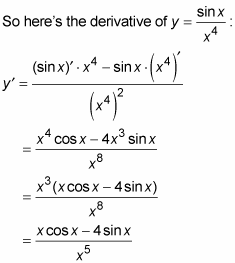##### Differential Equations For Dummies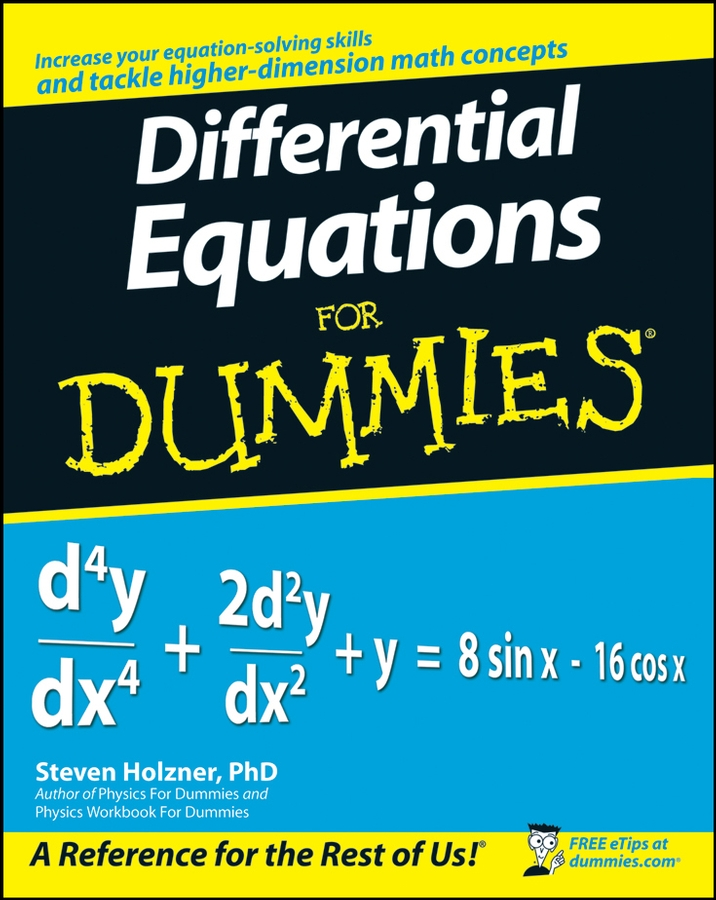There are special rules for finding the derivative of the product of two functions or the quotient of two functions; these are the product rule and the quotient rule, respectively.

• Product rule: You use the product rule for — hold on to your hat — the product of two functions like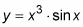Here's the basic product rule: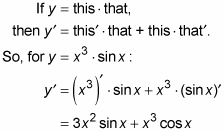• Quotient rule: You can probably guess what this rule is for — the quotient of two functions like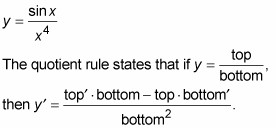The trick to using this rule is knowing the order of the terms in the numerator. Think of it like this: You’re doing a derivative, so the first thing you do is to take a derivative. And is it more natural to begin at the top or the bottom of a fraction? The top, of course. So the quotient rule begins with the derivative of the top. If you remember that, the rest of the numerator is almost automatic. Also note that the numerator is exactly like the product rule except for the subtraction sign. Focus on these points and you’ll remember the quotient rule ten years from now — oh, sure. (By the way, if you’re using a textbook, it probably gives this rule in an equivalent but slightly different form that is harder to memorize. You should memorize it the way it is presented here. It’s the easier and better way to learn it.)